# 【排序】--C语言实现快速排序

## 快速排序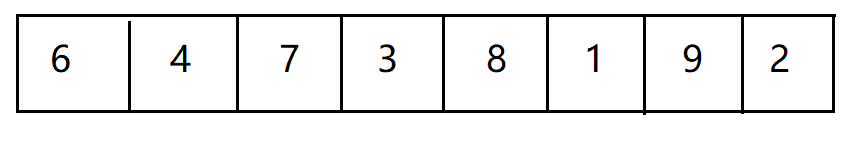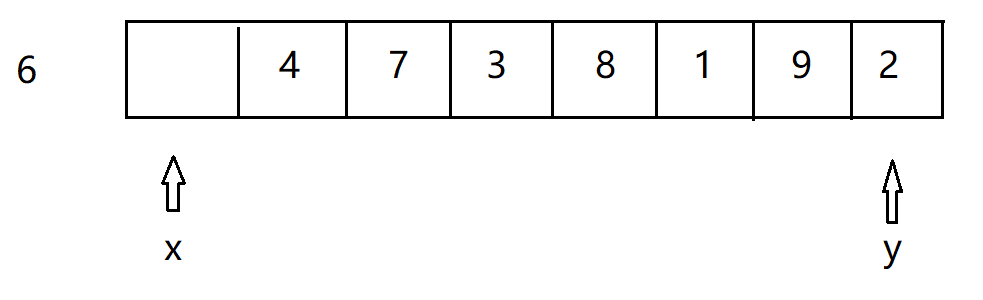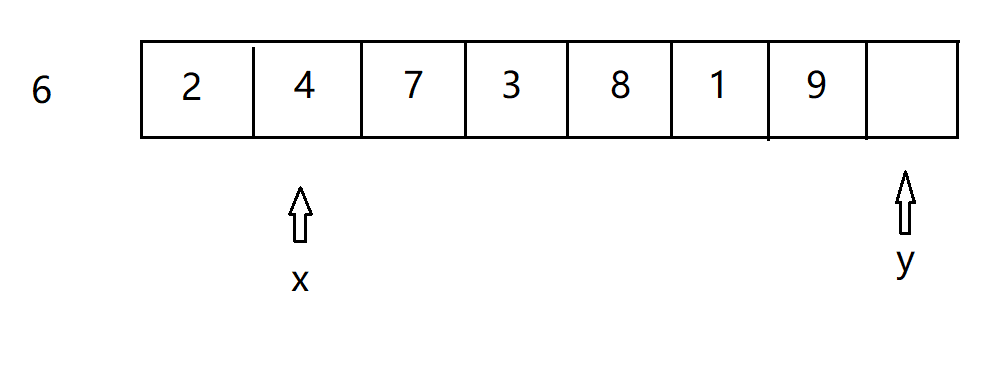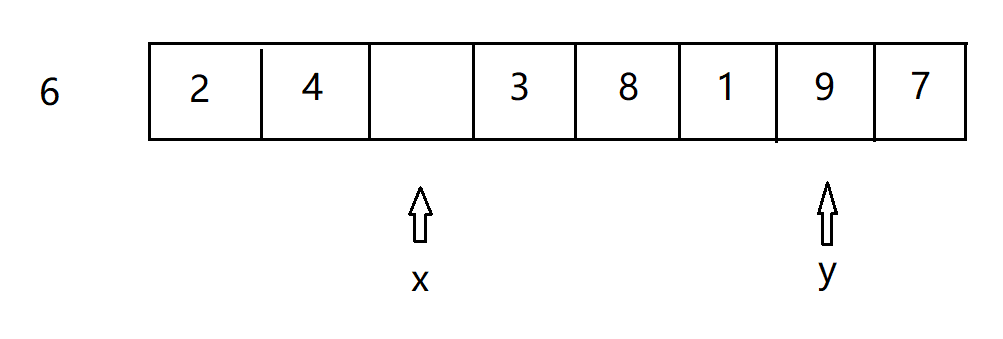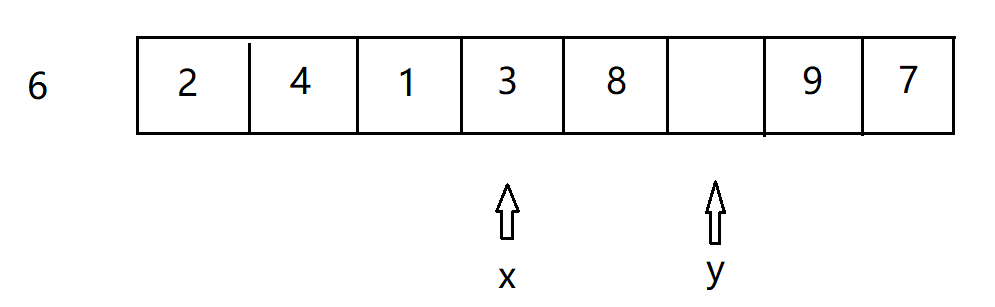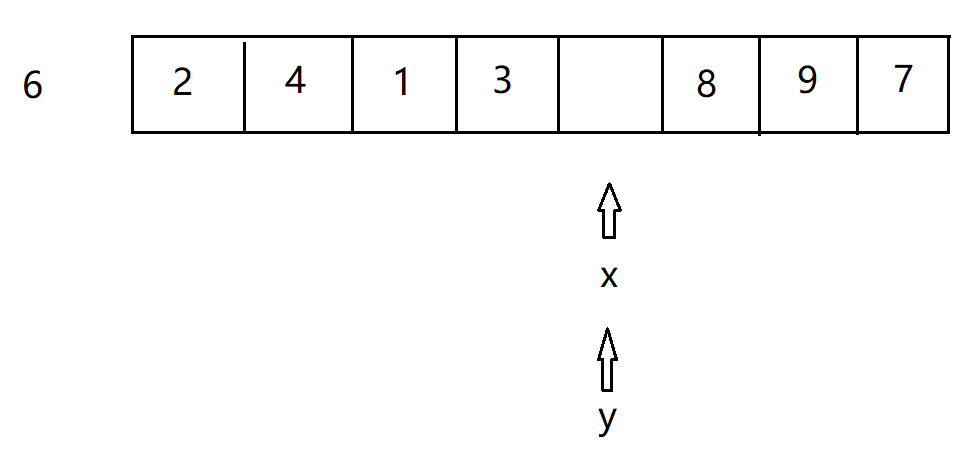## 代码

#include <stdio.h>

void QuickSort(int *a, int start, int end)
{
if (start >= end)
{
return;
}

int x = start;
int y = end;
int base = a[start];

while (x < y)
{
while (a[y] > base && x < y)
{
y--;
}

if (x < y)
{
a[x++] = a[y];
}

while (a[x] < base && x < y)
{
x++;
}

if (x < y)
{
a[y--] = a[x];
}
}
a[x] = base;

QuickSort(a, start, x - 1);
QuickSort(a, x + 1, end);
}

int main()
{
int i;
int array = {9, 3, 4, 4, 6, 0, 1, 3, 8, 5};

QuickSort(array, 0, sizeof(array) / sizeof(array) - 1);
for (i = 0; i < sizeof(array) / sizeof(array); i++)
{
printf("%d ", array[i]);
}
printf("\n");

return 0;
}05-08
03-1813805-262万+
02-06160
07-02
11-0410万+
05-292274
12-04
03-31
09-254708